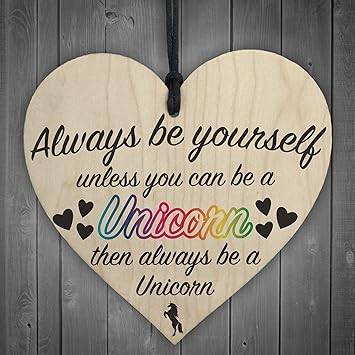# Day 4: The Architect

• Wood = 1

Clay = 8

Stone = 5

185+185+185=555

• Lumber - 1

Clay - 8

Iron - 5

Server: Travian COM 3 (TS3)

• Wood = 1

Clay = 8

Stone = 5

Anaethema on COM3

• Iron is 5 u got 3x5=15 its a up down sum so u write 5 and 1 goes up to next digit sum cause of that Iron is 5 only number that 3x of it result in a number with first right digit would be same as the number.

Bricks or Clay is 8 cause 3x8=24 and +1 from previous sum u get 25 which u write down the 5 here Iron 2 goes up for next digits sum

Lumber or Wood is 1 cause 3x1=3 and +2 from previous sum u get 5 which u write down 5 the Iron

and the answer of the sum is 555 hence 3 Irons

Bahramchoobin

from: ts15.anglosphere.travian.com

• Lumber - 1

Clay - 8

Iron - 5

https://ts15.travian.com

XOPBAT ru6 (eL norte) 2009 / Leonor ru1 (I.F.F) 2008 / Sinzoky ru4 (system) 2010 /
XOPBAT tx2(Greens) 2012 / Fruits ЧМ (OstF FF) 2012 / OSA rux16 [M] 2013 / Пчелки ru8 (Ракшасы) 2014• The Anwser is : 555}~{ wood:1 clay:8 iron:5

185
185
+ 185
_____
555

Nickname: Matt
Server: ts15.travian.com

• cammo

ts15.travian.ro

(x) wood= 1

(y) clay= 8

(z) iron= 5

solution:

z multiplied by 3 must end in the same number: possible values: 0,5

if (z) iron= 0, than the sum value zzz=0 and not 000 - not a viable answer

id (z) iron= 5, the sum is 555=(xyz)*3

555/3=185

cammo, ts15.travian.ro

• Nickname = usual

Server = com 15 ( codex victoria)

Number is 1 8 5..that is wood = 1, clay = 8, and iron = 5...

Wood clay iron+ wood clay iron + wood clay iron = iron iron iron

So

185+185+185 = 555

• 3 multiplied by a number is a different number.

the last digit (iron) multiplied by 3 must have the last digit to also be itself. the only number (from 0-9) that acheives this is 5. therefore iron = 5.

therefore the big number = 555. since the 3 numbers added to get to 555 are the same, we can simply divide 555 by 3. We get 185.

therefore:

wood = 1
clay = 8

iron = 5.

acc: Climate Change

server ts15.com codexV• Wood: 1

Clay: 8

Iron: 5

185 + 185 + 185 = 555

Name: DeLongest

Server: COMX

• mohamed gomaa

/ts15.travian.com/

185

185

185

555

BASTARDOS SIN GLORIA

codex Victoria

• Mjölnir - groupint.travian.com

If we note

wood = A

clay = B

iron = C

our problem resumes to

ABC+ABC+ABC=CCC

“ABC” is a 3 digit number. Then, each one of them, i.e. A,B,C are 3 digits.

The sum of 3 C’s in the unit’s place again results in C. This is possible only when “C” is either 5 or 0.

Case 1: C=0

In this case each of the digit accounts to zero. That means A,B,C is 0. (trivial answer, not pretty much expected)

Case2: C=5

In this case

AB5+AB5+AB5=555 => AB5=555/3 => AB5=185 =>

A=1

B-8

C-5

• 185

185

+185

-----------

555= 1= 8= 5

Flea

Ts15.travian.com

Wood = 1

Clay = 8

Iron = 5

• So here,

Wood represents number 1

Clay represents number 8

Iron represents number 5

So, Equation will be as follows::

185

185

+185

=555

IGN:- Poison

Server:- Speed 3x